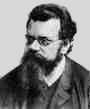Home

Lecture Notes

PHY 418: Statistical Mechanics I
Prof. S. Teitel stte@pas.rochester.edu ---- Spring 2012

## Lecture Notes

The lecture note files correspond roughly to the material presented in a given day's lecture. But you may on occassion find the end of one day's lecture at the start of the file for the next day's lecture, so please look there if you think there might be something missing.

• Lecture 0 - Sketching the history of statistical mechanics and thermodynamics (another such page here.)

• Lecture 1 - Postulates of classical thermodynamics, entropy, temperature, pressure, chemical potential, condition for thermal equilibrium

• Lecture 2 - Conditions for mechanical and chemical equilibrium, concavity of the entropy, equations of state, Euler relation, Gibbs-Duhem relation

• Lecture 3 - Entropy of the ideal gas, entropy maximum vs energy minimum principle, Legendre transformation

• Lecture 4 - Helmholtz and Gibbs free energies, enthalpy, the grand potential, reservoirs, extremum principles for these new thermodynamic potentials

• Lecture 5 - Maxwell relations, response functions and relations between them

• Lecture 6 - Curvature of free energies and stability requirements for response functions, kinetic theory of ideal gas, Maxwell velocity distribution

Lecture 6 supplement - Heat engines, thermodynamic efficiency, Carnot cycle
• Lecture 7 - Ergodic hypothesis, statisitcal ensembles, density matrix, Liouville's theorem, microcanonical ensemble, density of states

• Lecture 8 - Density of states and connection to entropy, entropy of the ideal gas and problem of extensivity, entropy of mixing and Gibbs' paradox

• Lecture 9 - Entropy of mixing continued, indistinguishable particles, the canonical ensemble and the canonical partition function

• Lecture 10 - Helholtz free energy and the canonical partition function, energy fluctuations, equivalence of canonical and microcanonical ensembles in the thermodynamic limit

• Lecture 11 - Average energy vs most probably energy, Stirling's formula, factoring the canonical partition function for non-interacting objects, Maxwell velocity distribution revisited

• Lecture 12 - The virial theorm, the equipartition theorem, elastic vibrations of a solid and the law of Dulong and Petit, paramagnetism of classical spins and the Curie law of paramagnetic susceptibility

• Lecture 13 - Entropy and information

• Lecture 14 - The grand canonical ensemble: the grand canonical partition function and the grand potential, fluctuations in the number of particles

• Lecture 15 - Fluctuations in the grand canonical ensemble continued, the grand canonical partition function for non-interacting particles, chemical equilibrium, a gas in equilibrium with a surface of absorption sites

• Lecture 16 - A gas in equilibrium with an absorbing surface, quantum ensembles, density matrix

• Lecture 17 - Quantum microcanonical, canonical, and grand canonical ensembles, quantum harmonic osciallator at temperature T, Bose-Einstein and Fermi-Dirac statistics, Pauli exlusion principle, occupation number representation, density matrix for two non-interacting particles

• Lecture 18 - Two particle density matrix continued, quantum correlations as an effective classical pair interaction, N-particle partition function in the position-space basis, partition functions for non-interacting quantum ideal gas

• Lecture 19 - Classical partition function in the occupation number representation, average occupation number, the classical vs quantum limits of the ideal gas, the quantized harmonic oscillator as bosons

• Lecture 20 - Debye model for the specific heat of a solid, black body radiation

• Lecture 21 - The quantum ideal gas, standard functions, pressure, density, energy, the leading correction to the classical limit

• Lecture 22 - The degenerate Fermi gas, ground state energy, the Sommerfeld expansion, chemical potential at finite temperature, specific heat, Pauli paramagnetism

• Lecture 23 - Pauli paramagnetism continued, ideal Bose gas

• Lecture 24 - Bose-Einstein condensation in an ideal gas of free bosons

• Lecture 25 - BEC in laser cooled atomic gases, classical spin models, constant magnetization vs constant magnetic field ensembles

• Lecture 26 - Broken symmetry and the possibility for a phase transition in the thermodynamic limit, Ising phase diagram

• Lecture 27 - Mean-field solution of the Ising model, critical exponents

• Lecture 28 - Mean-field solution and critical exponents continued, Maxwell construction, Landau theory of phase transitions, the liquid-gas transition

Lecture 29 - Landau theory continued, critical exponents, specific heat, exact solution of one dimensional Ising model

• Lecture 30 - Fluctuations and diverging length scale at a 2nd order critical point, Landau-Ginzburg theory, correlation functions, upper and lower critical dimensions